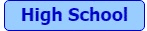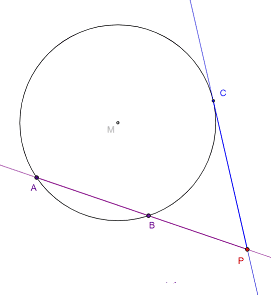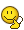# Math Challenge - August 2020

• Challenge
• Featured
Mentor
1. (solved by @nuuskur ) Let ##K## be a non-empty compact subset of ##\Bbb{C}##. Construct a bounded operator ##u: H \to H## on some Hilbert space ##H## that has spectrum ##\sigma(u) =K##. (MQ)

2. Let ##f,g:[0,2]\to\mathbb{R}## be continuous functions such that ##f(0)=g(0)=0## and ##f(2)=g(2)=2##. Show that there exist ##a,b\in [0,2)\,,a\neq b\,.## such that ##f(a)-f(b)## and ##g(a)-g(b)## are both integers. (IR)

3. (solved by @nuuskur ) Let ##V## be a normed vector space and ##W## be a closed linear subspace. If ##V/W## is a Banach space for the quotient norm, show that ##V## is a Banach space as well. (MQ)

4. (solved by @nuuskur ) Let ##(\Omega, \mathcal{F}, \Bbb{P})## be a probability space and ##X: \Omega \to \Bbb{R}## be a random variable. If ##\Bbb{P}(X \in A) \in \{0,1\}## for all Borel sets ##A##, show that there is a constant ##c \in \Bbb{R}## such that ##\Bbb{P}(X= c) = 1## (i.e. ##X## is constant almost surely). (MQ)

5. Let ##X,Y## be Banach spaces over the field ##\mathbb{R}## or ##\mathbb{C}##. By ##B_X(\hat x,R)## we denote the open ball of this space:
$$B_X(\hat x,R)=\{x\in X\mid \|x-\hat x\|_X<R\},$$ and ## \overline B_X(\hat x,R)=\{x\in X\mid \|x-\hat x\|_X\le R\}.##
Consider a continuous bilinear function ##A:X\times X\to Y## such that
$$\|A(x_1,x_2)\|_Y\le a\|x_1\|_X\|x_2\|_X,\quad A(x_1,x_2)=A(x_2,x_1).$$
Let ##B:X\to Y## stand for a bounded linear operator onto:
$$\overline B_Y(0,b)\subseteq B(\overline B_X(0,1))$$ with some positive constant ##b##. Let ##C## stand for a fixed element of ##Y,\quad \|C\|_Y=c##.
Show that
If ##D=b^2-4ac\ge 0## then the equation
$$A(x,x)+Bx+C=0$$ has a solution. (WR)

6. (solved by @nuuskur ) Show that in ZF the Tychonoff theorem (product of compact spaces is compact) implies the axiom of choice. (MQ)

7. (solved by @nuuskur ) Let ##f: (X,d_X) \to (Y,d_Y)## be a map between metric spaces (not necessarily continuous!). Show that ##D(f):= \{x \in X\mid f \mathrm{\ is \ not \ continuous \ at \ x}\}## is a Borel-measurable set of ##X##. (MQ)

8. (solved by @benorin , @Fred Wright , and @nuuskur ) Let ##F## be a meromorphic function (holomorphic up to isolated poles) in ##\mathbb{C}## with the following properties:
(1) ##F## is holomorphic (complex differentiable) in the half plane ##H(0)=\{z\in \mathbb{C}\, : \,\Re(z)>0\}.##
(2) ##zF(z)=F(z+1).##
(3) ##F## is bounded in the strip ##\{z\in \mathbb{C}\, : \,1\leq\Re(z)\leq 2\}.##
Show that ##F(z)=F(1)\Gamma(z).## (FR)

9. (solved by @PeroK ) Show that there exists no continuous function ##g: \Bbb{C}\setminus \{0\}\to \Bbb{C}## such that ##e^{g(z)} = z## for all ##z\in \Bbb{C}\setminus \{0\}## (i.e. there is no continuous logarithm on ##\Bbb{C}\setminus \{0\})##. (MQ)
(Corrected version, credits go to @PeroK for pointing out and solving the original problem.)

10. (solved by @PeroK ) Show that if ##f## is any continuous real function and ##n## any positive number (FR),
$$I:=\int_{n^{-1}}^{n} f\left(x+\dfrac{1}{x}\right)\,\dfrac{\log x}{x}\,dx =0.$$High Schoolers only

11.
(solved by @Not anonymous ) Let ##a<b<c<d## be real numbers. Sort ##x=ab+cd\, , \,y=bc+ad\, , \,z=ac+bd## and prove it.

12. (solved by @Not anonymous ) Prove ##\overline{CP}^2=\overline{AP}\cdot \overline{BP}\,.##13. How big is the probability for two pocket aces in Texas Hold'em? Assume we have seen a show down in a heads-up. How many possible combinations are there, how many combinations of possible starting hands can the opponents have? How many possible community cards?

14. (solved by @etotheipi ) Everybody knows that Schrödinger's cat is trapped in the box since ##1935.## Not well known is the fact, that the radioactive material was ten ##{}^{14}C## isotopes. Calculate the probability that the cat is still alive.

15. (solved by @ItsukaKitto ) Show that there is no rational solution for ##p^2+q^2+r^2=7.##

Last edited:
•••Isaac0427, ItsukaKitto, DifferentialGalois and 8 others

## Answers and Replies

etotheipi
14. Everybody knows that Schrödinger's cat is trapped in the box since ##1935.## Not well known is the fact, that the radioactive material was ten ##{}^{14}C## isotopes. Calculate the probability that the cat is still alive.

The time of an individual decay (which is independent of any other decays) follows an exponential distribution ##T \sim \text{Exp}(\lambda)## with ##P(T > t) = e^{-\lambda t}##. The number of atoms out of 10 that have decayed, ##X##, follows a binomial distribution ##X \sim \text{B}(10, 1-e^{-\lambda t})##, so $$P(X=0) =e^{-10\lambda t}$$The half life of ##^{14}\text{C}## is ##t_{1/2} = 5700 \text{ years}##, so ##\lambda t = \frac{\ln{2}}{5700 \text{ years}} \times 85 \text{ years} = \frac{17 \ln{2}}{1140}## which gives, assuming the Geiger counter detects all radiation, ##P(X=0) \approx0.90##

But given the maximum lifespan of a cat is only about 16 years...

Last edited by a moderator:
••DifferentialGalois, benorin, Wrichik Basu and 1 other person
etotheipi
10. Show that if ##f## is any continuous real function and ##n## any positive number (FR),
$$I:=\int_{n^{-1}}^{n} f\left(x+\dfrac{1}{x}\right)\,\dfrac{\log x}{x}\,dx =0.$$

Let ##u = x + \frac{1}{x}##, then ##x = \frac{u \pm \sqrt{u^2-4}}{2} := \alpha(u)## and$$I = \int_{n + n^{-1}}^{n + n^{-1}} f(u) \frac{\log(\alpha(u))}{\alpha(u)(1-\frac{1}{\alpha^2(u)})} du = 0$$because the limits are equal...
2. Let ##f,g:[0,2]\to\mathbb{R}## be continuous functions such that ##f(0)=g(0)=0## and ##f(2)=g(2)=2##. Show that there exist ##a,b\in [0,2)## such that ##f(a)-f(b)## and ##g(a)-g(b)## are both integers. (IR)

Doesn't ##a = b## work?

Last edited by a moderator:
Infrared
Science Advisor
Gold Member
@etotheipi Fair enough, but the intent was to show that it can be done with ##a\neq b##.

•etotheipi
PeroK
Science Advisor
Homework Helper
Gold Member
2020 Award
Let ##x = \frac{1}{u}##.
$$I = \int_{n}^{1/n} f(u + \frac 1 u) (-\log(u))u(-\frac{1}{u^2})du = \int_{n}^{1/n} f(u + \frac 1 u) \frac{\log(u)}{u}du = -I$$
Hence ##I = 0##

PeroK
Science Advisor
Homework Helper
Gold Member
2020 Award
Let ##u = x + \frac{1}{x}##, then ##x = \frac{u \pm \sqrt{u^2-4}}{2} := \alpha(u)## and$$I = \int_{n + n^{-1}}^{n + n^{-1}} f(u) \frac{\log(\alpha(u))}{\alpha(u)(1-\frac{1}{\alpha^2(u)})} du = 0$$because the limits are equal...
The new variable ##u## is not on a single interval for ##x \in [1/n, n]##.

•etotheipi
PeroK
Science Advisor
Homework Helper
Gold Member
2020 Award
14. Everybody knows that Schrödinger's cat is trapped in the box since ##1935.## Not well known is the fact, that the radioactive material was ten ##{}^{14}C## isotopes. Calculate the probability that the cat is still alive.

A cat's been trapped in a box for 85 years and you think it might still be alive?

••DifferentialGalois, member 587159, nuuskur and 1 other person
etotheipi
9. Show that there exists no continuous function ##g: \Bbb{C}\setminus \{0\}\to \Bbb{C}## such that ##g(e^{z}) = z## for all ##z\in \Bbb{C}\setminus \{0\}## (i.e. there is no continuous logarithm on ##\Bbb{C}\setminus \{0\})##. (MQ)

This could be ridiculously along the wrong lines, but... let ##z = a + bi##, so $$g(e^z) = z$$ $$g(ie^a \sin{b} + e^a \cos{b}) = a + bi$$Since ##e^z = ie^a \sin{b} + e^a \cos{b}##, then ##\text{Re}(e^z) = e^a \cos{b}## and ##\text{Im}(e^z) = e^a \sin{b}##. This means that ##\text{Re}(e^z)^2 + \text{Im}(e^z)^2 = (e^a)^2 (\sin^2 b + \cos^2 b) = (e^a)^2##. We end up with$$a = \ln{\sqrt{\text{Re}(e^z)^2 + \text{Im}(e^z)^2}}$$and$$b = \arctan{\left(\frac{\text{Im}(e^z)}{\text{Re}(e^z)} \right)} + n\pi$$So$$g(e^z) = \ln{\sqrt{\text{Re}(e^z)^2 + \text{Im}(e^z)^2}} + i \left(\arctan{\left(\frac{\text{Im}(e^z)}{\text{Re}(e^z)} \right)} + n\pi \right)$$but this isn't a function, because it's not possible to recover the specific ##n## that corresponds to ##b##?

Last edited by a moderator:
PeroK
Science Advisor
Homework Helper
Gold Member
2020 Award
13. How big is the probability for two pocket aces in Texas Hold'em? Assume we have seen a show down in a heads-up. How many possible combinations are there, how many combinations of possible starting hands can the opponents have? How many possible community cards?

Is there an explanation of this for non-poker-playing high-schoolers?

•DifferentialGalois
member 587159
This could be ridiculously along the wrong lines, but... let ##z = a + bi##, so $$g(e^z) = z$$ $$g(ie^a \sin{b} + e^a \cos{b}) = a + bi$$Since ##e^z = ie^a \sin{b} + e^a \cos{b}##, then$$a = \ln{\sqrt{\text{Re}(e^z)^2 + \text{Im}(e^z)^2}}$$and$$b = \arctan{\left(\frac{\text{Im}(e^z)}{\text{Re}(e^z)} \right)} + n\pi$$so we could say...$$g(z) = \ln{\sqrt{\text{Re}(e^z)^2 + \text{Im}(e^z)^2}} + i \left(\arctan{\left(\frac{\text{Im}(e^z)}{\text{Re}(e^z)} \right)} + n\pi \right)$$but this isn't a function, because it's not possible to recover the specific ##n## that corresponds to ##b##?

How do you get that expression for the value of ##a##?

PeroK
Science Advisor
Homework Helper
Gold Member
2020 Award
The complex exponential function is not one-to-one, so cannot have an inverse, continuous or otherwise.

We have: $$e^z = e^x(\cos y + i\sin y)$$
Consider ##z_1 = i\frac\pi 2## and ##z_2 = i\frac{5\pi}{2}##, then
$$e^{z_1} = e^{z_2} = i$$

•DifferentialGalois, member 587159 and etotheipi
etotheipi
How do you get that expression for the value of ##a##?

I just used that ##\text{Re}(e^z) = e^a \cos{b}## and ##\text{Im}(e^z) = e^a \sin{b}## so ##\text{Re}(e^z)^2 + \text{Im}(e^z)^2 = (e^a)^2 (\sin^2 b + \cos^2 b) = (e^a)^2##

member 587159
The complex exponential function is not one-to-one, so cannot have an inverse, continuous or otherwise.

We have: $$e^z = e^x(\cos y + i\sin y)$$
Consider ##z_1 = i\frac\pi 2## and ##z_2 = i\frac{5\pi}{2}##, then
$$e^{z_1} = e^{z_2} = i$$

Your attempt made me realise that I meant to ask for the non-existence of a continuous function ##g:\Bbb{C}\setminus \{0\}\to \Bbb{C}## with ##\exp(g(z))=z## for all ##z\in \Bbb{C}\setminus \{0\}##.

Since ##\exp: \Bbb{C}\to \Bbb{C}\setminus\{0\}## is surjective, a set-theoretical right inverse exists but your task is to prove it is not continuous.

Sorry for the inconvenience.
But we are honest and you'll get credit for the attempt but the question remains open.

•DifferentialGalois
member 587159
I just used that ##\text{Re}(e^z) = e^a \cos{b}## and ##\text{Im}(e^z) = e^a \sin{b}## so ##\text{Re}(e^z)^2 + \text{Im}(e^z)^2 = (e^a)^2 (\sin^2 b + \cos^2 b) = (e^a)^2##

Add all these details in your attempt so it is easier for me to identify the mistake :) While the question was flawed due to my mistake, I do want to clear out where you were going wrong so we can learn something about it :)

•etotheipi
Compactness is equivalent to finite intersection property (FIP). Cf Proposition 3.1
Let $X_\alpha,\ \alpha\in I$, be a non-empty family of non-empty sets. We show $\prod_{\alpha\in I} X_\alpha \neq\emptyset$ (AC). Suppose $z\notin \bigcup _{\alpha\in I} X_\alpha$ (the union is a set by ZF). Equip $X_\alpha \cup \{z\}$ with cofinite topology, $\alpha\in I$. These are compact. By Tikhonov's theorem $S:=\prod_{\alpha\in I} X_\alpha\cup\{z\}$ is compact (under product topology). Fix $\alpha\in I$. The canonical projection $\pi_\alpha :S\to X_\alpha\cup\{z\}$ is continuous. Since $X_\alpha \subset X_\alpha\cup\{z\}$ is closed ($\{z\}$ is open), the preimage $\pi_\alpha^{-1}(X_\alpha) \subseteq S$ is closed. We show this family of preimages has FIP. Take $n\in\mathbb N$ and let $x_{\alpha_j} \in X_{\alpha _j},\ 1\leq j\leq n$. We then have
$$s(\alpha) := \begin{cases} x_{\alpha _j}, &\text{if }\alpha = \alpha _j \\ z, &\text{otherwise} \end{cases} \Rightarrow s\in \bigcap _{k=1}^n \pi_{\alpha_k}^{-1}(X_{\alpha _k}).$$
Due to compactness of $S$ there exists $y\in S$ such that $y\in \bigcap _{\alpha\in I} \pi _{\alpha}^{-1}(X_\alpha)$ (because the family of preimages has FIP). By definition $\pi _\alpha (y)\in X_\alpha$ for every $\alpha\in I$, thus $y\in\prod _{\alpha\in I}X_\alpha$.
Fun fact. Tikhonov's theorem is equivalent to the axiom of choice.

Last edited:
•member 587159
PeroK
Science Advisor
Homework Helper
Gold Member
2020 Award
Your attempt made me realise that I meant to ask for the non-existence of a continuous function ##g:\Bbb{C}\setminus \{0\}\to \Bbb{C}## with ##\exp(g(z))=z## for all ##z\in \Bbb{C}\setminus \{0\}##.

Since ##\exp: \Bbb{C}\to \Bbb{C}\setminus\{0\}## is surjective, a set-theoretical right inverse exists but your task is to prove it is not continuous.

Sorry for the inconvenience.
But we are honest and you'll get credit for the attempt but the question remains open.
That's perhaps getting beyond my knowldege. It's not hard to show that the inverse must take the form:
$$g(z) = \ln r + i\theta$$
Which has a discontinuity (branch cut) along the positive x-axis. Is the problem to show that there must be a branch cut somewhere?

etotheipi
Add all these details in your attempt so it is easier for me to identify the mistake :) While the question was flawed due to my mistake, I do want to clear out where you were going wrong so we can learn something about it :)

Whoops, the last line is supposed to read ##g(e^z)##, not ##g(z)##. Then I end up with what we'd expect for a logarithm, that ##g(z) = \ln{\sqrt{x^2+y^2}} + i\arctan{\frac{y}{x}}##, except I just took a very convoluted routemember 587159
Suppose we had $g:\mathbb C^* \to \mathbb C$ with $g(e^z) = z$ continuous. It would be a continuous inverse to $z\mapsto e^z,\ z\in\mathbb C.$ Thus $\mathbb C\cong \mathbb C^*$, but that's impossible. The rough explanation is: homemorphism means same number of holes. $\mathbb C^*$ has a hole in it, so it can't be homemorphic to $\mathbb C$.

Complex exponential isn't injective, any way, so a contradiction is arrived at earlier, even.

As stated in an earlier post, my question was flawed: see post #13. Sorry for the inconvenience, I'm waiting for post 1 to be edited.

member 587159
I'm confused. In #13 you asked for a proof that no continuous logarithm exists. Didn't I achieve that?

The question should be ##\exp(g(z))=z## not ##g(\exp(z))=z## as in your attempt.

member 587159
Compactness is equivalent to finite intersection property (FIP). Cf Proposition 3.1
Let $X_\alpha,\ \alpha\in I$, be a non-empty family of non-empty sets. We show $\prod_{\alpha\in I} X_\alpha \neq\emptyset$ (AC). Suppose $z\notin \bigcup _{\alpha\in I} X_\alpha$ (the union is a set by ZF). Equip $X_\alpha \cup \{z\}$ with cofinite topology, $\alpha\in I$. These are compact. By Tikhonov's theorem $S:=\prod_{\alpha\in I} X_\alpha\cup\{z\}$ is compact (under product topology). Fix $\alpha\in I$. The canonical projection $\pi_\alpha :S\to X_\alpha\cup\{z\}$ is continuous. Since $X_\alpha \subset X_\alpha\cup\{z\}$ is closed ($\{z\}$ is open), the preimage $\pi_\alpha^{-1}(X_\alpha) \subseteq S$ is closed. We show this family of preimages has FIP. Take $n\in\mathbb N$ and let $x_{\alpha_j} \in X_{\alpha _j},\ 1\leq j\leq n$. We then have
$$s(\alpha) := \begin{cases} x_{\alpha _j}, &\text{if }\alpha = \alpha _j \\ z, &\text{otherwise} \end{cases} \Rightarrow s\in \bigcap _{k=1}^n \pi_{\alpha_k}^{-1}(X_{\alpha _k}).$$
Due to compactness of $S$ there exists $y\in S$ such that $y\in \bigcap _{\alpha\in I} \pi _{\alpha}^{-1}(X_\alpha)$ (because the family of preimages has FIP). By definition $\pi _\alpha (y)\in X_\alpha$ for every $\alpha\in I$, thus $y\in\prod _{\alpha\in I}X_\alpha$.
Fun fact. Tikhonov's theorem is equivalent to the axiom of choice.

Looks correct! Well done!

member 587159
That's perhaps getting beyond my knowldege. It's not hard to show that the inverse must take the form:
$$g(z) = \ln r + i\theta$$
Which has a discontinuity (branch cut) along the positive x-axis. Is the problem to show that there must be a branch cut somewhere?

There are multiple approaches. First, you need to say what convention you use: I guess you write ##z=r e^ {i \theta}##. What domain do you allow for ##\theta##?

Then, why does ##g## take that form and why does it have a discontinuity?

member 587159
You're right, I can't read. I'm going to need some black magic for this i.e complex analysis. To be a bit nitpicky, when you say there is no continuous logarithm on $\mathbb C^*$ you actually mean there is no holomorphic map acting as a logarithm, because any logarithm is holomorphic.
Let $g: \mathbb C^* \to \mathbb C$ satisfy the identity $e^{g(z)} = z$. Then $g$ is holomorphic (Theorem 1.1). Also, @PeroK 's assertion is an immediate corollary (Cor 1.1). On the other hand, $g$ is a logarithm if and only if $g$ is a primitive for $\frac{1}{z}$ such that $e^{g(w)} = w$ for some $w\in\mathbb C^*$. By Cauchy's integral theorem there cannot exist such a primitive for $\frac{1}{z}$ on $\mathbb C^*$. Indeed, otherwise we would have
$$0=\oint_{|z|=1} g'(z) dz = \oint_{|z|=1} \frac{1}{z} dz = 2i\pi.$$

But the plane ##\Bbb{C}## with the origin removed is not simply connected (consider fundamental group), so the theorem does not apply. Also, if the plane was simply connected your theorem would contradict the exercise.

But the plane ##\Bbb{C}## with the origin removed is not simply connected (consider fundamental group), so the theorem does not apply. Also, if the plane was simply connected your theorem would contradict the exercise.
Correct. My bad.

Mentor
The time of an individual decay (which is independent of any other decays) follows an exponential distribution ##T \sim \text{Exp}(\lambda)## with ##P(T > t) = e^{-\lambda t}##. The number of atoms out of 10 that have decayed, ##X##, follows a binomial distribution ##X \sim \text{B}(10, 1-e^{-\lambda t})##, so $$P(X=0) =e^{-10\lambda t}$$The half life of ##^{14}\text{C}## is ##t_{1/2} = 5700 \text{ years}##, so ##\lambda t = \frac{\ln{2}}{5700 \text{ years}} \times 85 \text{ years} = \frac{17 \ln{2}}{1140}## which gives, assuming the Geiger counter detects all radiation, ##P(X=0) \approx0.90##

But given the maximum lifespan of a cat is only about 16 years...
##5730## years, but this doesn't affect the answer very much..

••etotheipi and nuuskur
member 587159
This could be ridiculously along the wrong lines, but... let ##z = a + bi##, so $$g(e^z) = z$$ $$g(ie^a \sin{b} + e^a \cos{b}) = a + bi$$Since ##e^z = ie^a \sin{b} + e^a \cos{b}##, then ##\text{Re}(e^z) = e^a \cos{b}## and ##\text{Im}(e^z) = e^a \sin{b}##. This means that ##\text{Re}(e^z)^2 + \text{Im}(e^z)^2 = (e^a)^2 (\sin^2 b + \cos^2 b) = (e^a)^2##. We end up with$$a = \ln{\sqrt{\text{Re}(e^z)^2 + \text{Im}(e^z)^2}}$$and$$b = \arctan{\left(\frac{\text{Im}(e^z)}{\text{Re}(e^z)} \right)} + n\pi$$So$$g(e^z) = \ln{\sqrt{\text{Re}(e^z)^2 + \text{Im}(e^z)^2}} + i \left(\arctan{\left(\frac{\text{Im}(e^z)}{\text{Re}(e^z)} \right)} + n\pi \right)$$but this isn't a function, because it's not possible to recover the specific ##n## that corresponds to ##b##?

Yes, the problem I see with this is that for different choices of ##z## you can have different values of ##n##. So you should write ##n= n(z)##.

•etotheipi